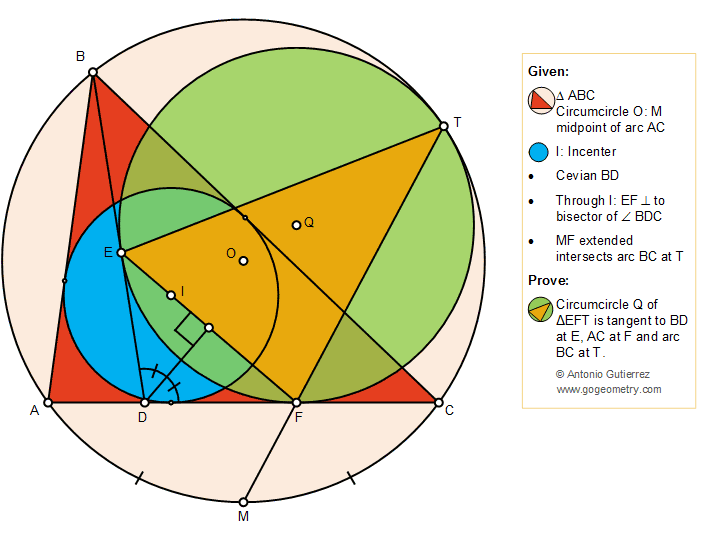## Sunday, February 24, 2019

### Geometry Problem 1417: Triangle, Cevian, Circumcircle, Incenter, Perpendicular, Angle Bisector, Tangent Circles

Geometry Problem. Post your solution in the comment box below.
Level: Mathematics Education, High School, Honors Geometry, College.

Details: Click on the figure below.#### 1 comment:

1.B,I and M are colinear, draw line BIM.
We have AM=CM=MI and MI^2=MF.MT.
Hence MI is tangent to circumcircle of tr.IFT.
We have Angle MIF = Angle MTI
Lets assume Angle ABD=x, then Angle DEF= 90-A/2 - x/2.
Also Angle MIF = Angle MTI = Angle BIE = 90 - A/2 -x/2 -[(B/2) - x] = C/2 + x/2
Angle BTI= Angle BTM - Angle MTI = C + B/2 - (C/2 + x/2)=C/2+B/2 -x/2=90 -A/2 -x/2=Angle DEF
Since Angle BTI = Angle DEF, quad.BEIT is cylic.

We get Angle EBI = Angle ETI = B/2 -x
Angle ETF = Angle ETI + Angle FTI = B/2 -x + C/2 +x/2= B/2 + C/2 - x/2= 90 - A/2 - x/2 = Angle DEF = Angle DFE hence DE and DF are tangent to circle Q.
Also we have Angle IET= Angle Angle MBT=y
Then Angle FTQ=90-y=Angle MTO
It means T,Q and O are colinear, hence Circle Q and O touch at point T.